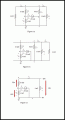# Circuit Analysis Chapter 2/ E2.23-Irwin

#### RBR1317

Joined Nov 13, 2010
706
As you can see, there are 2 nodes with unknown voltage. So write the 2 node equations and solve for V0 & V1.

#### Özbey

Joined Dec 7, 2018
12
As you can see, there are 2 nodes with unknown voltage. So write the 2 node equations and solve for V0 & V1.
Thank you so much. I solved it thanks to you.

#### MrAl

Joined Jun 17, 2014
10,082
Thank you so much. I solved it thanks to you.
Why does it say "V1=15v" near the bottom of the first post?
That does not seem to work out if the only source is the 20ma current source.
V0=60v does work out ok.
(See attachment where circuit complexity is reduced to make the solution more apparent)